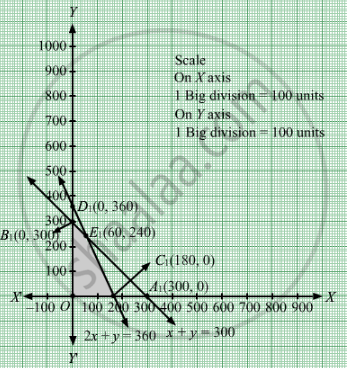# A Firm Manufactures Two Types of Products a and B and Sells Them at a Profit of Rs 5 per Unit of Type a and Rs 3 per Unit of Type B. - Mathematics

Sum

A firm manufactures two types of products A and B and sells them at a profit of Rs 5 per unit of type A and Rs 3 per unit of type B. Each product is processed on two machines M1 and M2. One unit of type A requires one minute of processing time on M1 and two minutes of processing time on M2, whereas one unit of type B requires one minute of processing time on M1 and one minute on M2. Machines M1 and M2 are respectively available for at most 5 hours and 6 hours in a day. Find out how many units of each type of product should the firm produce a day in order to maximize the profit. Solve the problem graphically.

#### Solution

Let x units of product A and y units of product B were manufactured.
Number of products cannot be negative.
Therefore, $x, y \geq 0$

According to question, the given information can be tabulated as

 Time on  $M_1$ (minutes) Time on  $M_2$ (minutes) Product A(x) 1 2 Product B(y) 1 1 Availability 300 360

The constraints are
$x + y \leq 300$ $2x + y \leq 360$

Firm manufactures two types of products A and B and sells them at a profit of Rs 5 per unit of type A and Rs 3 per unit of type B.Therefore, x units of product A and y units of product B costs Rs 5x and Rs 3y respectively.
Total profit = Z =  $5x + 3y$ which is to be maximised
Thus, the mathematical formulat​ion of the given linear programmimg problem is
Max Z= $5x + 3y$
subject to
$x + y \leq 300$ .
$2x + y \leq 360$
$x, y \geq 0$
First we will convert inequations into equations as follows:
x + y = 300, 2x + y = 360, x = 0 and y = 0

Region represented by x + y ≤ 300:
The line x + y = 300 meets the coordinate axes at A1(300, 0) and B1(0, 300) respectively. By joining these points we obtain the line
x + y = 30. Clearly (0,0) satisfies the x + y = 30. So, the region which contains the origin represents the solution set of the inequation
x + y ≤ 300.

Region represented by 2x y ≤ 360:
The line 2x + y = 360 meets the coordinate axes at C1(180, 0) and D1(0, 360) respectively. By joining these points we obtain the line
2x + y = 360. Clearly (0,0) satisfies the inequation 2x y ≤ 360. So,the region which contains the origin represents the solution set of the inequation 2x y ≤ 360.

Region represented by ≥ 0 and y ≥ 0:
Since, every point in the first quadrant satisfies these inequations. So, the first quadrant is the region represented by the inequations x ≥ 0, and y ≥ 0.

The feasible region determined by the system of constraints x + y ≤ 300, 2x y ≤ 360, x ≥ 0 and y ≥ 0 are as follows.The corner points are O(0, 0), B1(0, 300), E1(60, 240) and C1(180, 0).

The values of Z at these corner points are as follows

 Corner point Z= 5x + 3y O 0 B1 900 E1 1020 C1 900

The maximum value of Z is Rs 1020 which is attained at  B1$\left( 60, 240 \right)$

Thus, the maximum profit is Rs 1020 obtained when 60 units of product A and 240 units of product B were manufactured.
Concept: Graphical Method of Solving Linear Programming Problems
Is there an error in this question or solution?

#### APPEARS IN

RD Sharma Class 12 Maths
Chapter 30 Linear programming
Exercise 30.4 | Q 28 | Page 54